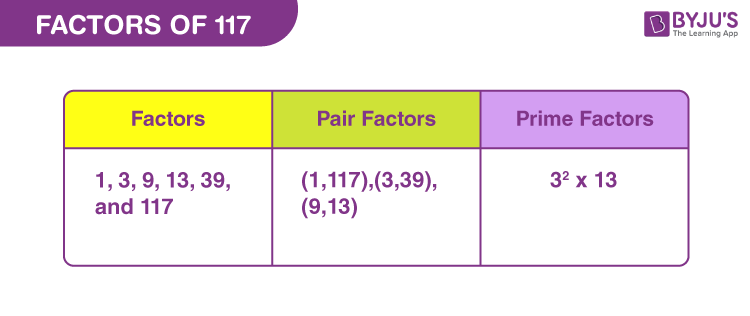# Factors of 117

In Mathematics, factors of 117 are the natural numbers that can uniformly divide the original number. In other words, if the product of two numbers equals 117, then the numbers are the required factors. 117 is an odd composite number and has more than two factors.

The factors are also called the divisors of 117. Let us find these factors here along with the pair factors. Also, find the prime factorization of 117 in this article.## How to Find the Factors of 117?

To find the factors of 117, we have to divide the original number by such natural numbers, so that the remainder is zero.

• 117 ÷ 1 = 117
• 117 ÷ 3 = 39
• 117 ÷ 9 = 13
• 117 ÷ 13 = 9
• 117 ÷ 39 = 3
• 117 ÷ 117 = 1

Therefore, there are a total sixteen factors of 117: 1, 3, 9, 13, 39 and 117.

## Pair Factors of 117

Pair factors are the integers, the product of which results in the original number. Therefore, the pair of factors of 117 are:

• 1 × 117 = 117
• 3 × 39 = 117
• 9 × 13 = 117

Therefore, the pair factors of 117 are (1, 117), (3, 39), (9, 13).

The negative pair factors can be written as the product of two negative numbers that result in 117.

• -1 × -117 = 117
• -3 × -39 = 117
• -9 × -13 = 117

Therefore, the negative pair factors of 117 are (-1, -117), (-3, -39), (-9, -13)

## Prime Factorisation of 117

The prime factorisation of 117 will result in a product form of prime factors of the original number. Thus, we get the prime factors of 117.

 117 ÷ 3 = 39 39 ÷ 3 = 13 13 ÷ 13 = 1

Therefore, the prime factors of 117 are 3 and 13. Thus, 117 is evenly divisible by 3 and 13.

 Prime factorisation of 117 = 3 x 3 x 13 Exponential form = 32 x 13

## Solved Examples

Q.1: What should be multiplied by 9 to get 117?

Solution: Suppose, n is the number to be multiplied by 9 to get 117.

So, n × 9 = 117

n = 117/9

n = 13

Therefore, 13 should be multiplied by 9 to get 117 as the result.

Q.2: What is the sum of factors of 117?

Solution: The factors of number 117 are 1, 3, 9, 13, 39, 117.

Sum of all the factors = 1 + 3 + 9 + 13 + 39 + 117

Sum = 182

Therefore, 182 is the required sum.

Q.3: What is the greatest common factor of 132 and 117?

Solution:

The factors of 117 are 1, 3, 9, 13, 39 and 117

Factors of 132 are 1, 2, 3, 4, 6, 11, 12, 22, 33, 44, 66, 132

Therefore, the greatest common factor of 132 and 117 is:

GCF (117, 132) = 3

### Practice Questions

1. What are the even divisors of 117?
2. Richard had 117 marbles and 9 marble pots. How many marbles can he keep in each marble pot?
3. Find the common factors of 117 and 127.
4. What is the area of tile with length 13 cm and width equal to 9 cm?
5. What is the GCF of 117 and 135?

## Frequently Asked Questions on Factors of 117

### What are the factors of 117?

The factors of 117 are 1, 3, 9, 13, 39 and 117.

### What two factors make 117?

The product of a pair of factors that make 117 are (1, 117), (3, 39), (9, 13).

### Is 117 a prime number?

117 is not a prime number, since it has factors greater than two.

### What is the GCF of 117 and 130?

The greatest common factor of 117 and 130 is 13.
13 x 9 = 117
13 x 10 = 130

### Is 117 a perfect square?

No, 117 is not a perfect square.

### What is the HCF of 117 and 221?

The HCF of 117 and 221 is 13.